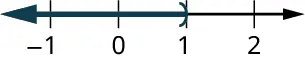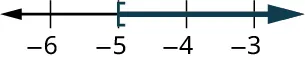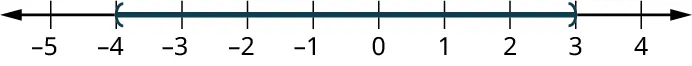Contemporary Mathematics

# 5.3Linear Inequalities in One Variable with Applications

Contemporary Mathematics5.3 Linear Inequalities in One Variable with Applications

Figure 5.5 These poll results, showing a margin of error at 4 percent, are an example of a real-world scenario that can be represented by linear inequalities.

### Learning Objectives

After completing this section, you should be able to:

1. Graph inequalities in one variable.
2. Solve linear inequalities in one variable.
3. Construct a linear inequality to solve applications.

In this section, we will study linear inequalities in one variable. Inequalities can be used when the possible values (answers) in a certain situation are numerous, not just a few, or when the exact value (answer) is not known but it is known to be within a range of possible values. There are many real-world scenarios that can be represented by linear inequalities. For example, consider the survey of the mayoral election in Figure 5.5 Surveys and polls are usually conducted with only a small group of people. The margin of error indicates a range of how the actual group of voters would vote given the results of the survey. This range can be expressed using inequalities.

Another example involves college tuition. Say a local community college charges $113 per credit hour. You budget$1,500 for tuition this fall semester. What are the number of credit hours that you could take this fall? Since this answer could be many different values, it can be expressed as an inequality.

### Graphing Inequalities on the Number Line

In Algebraic Expressions, we introduced equality and the $==$ symbol. In this section, we look at inequality and the symbols $<<$, $>>$, $≤≤$, and $≥≥$. The table below summarizes the symbols and their meaning.

Symbol Meaning
$<<$ less than
$>>$ greater than
$≤≤$ less than or equal to
$≥≥$ greater than or equal to

Suppose you had the inequality statement $x>3x>3$. What possible number or numbers would make the inequality $x>3x>3$ true? If you are thinking, "$xx$ could be 4," that's correct, but $xx$ could also be 5, 6, 37, 1 million, or even 3.001. The number of solutions is infinite; any number greater than 3 is a solution to the inequality $x>3x>3$.

Rather than trying to list all possible solutions, we show all the solutions to the inequality $x>3x>3$ on the number line. All the numbers to the right of 3 on the number line are shaded, to show that all numbers greater than 3 are solutions. At the number 3 itself, an open parenthesis is drawn, since the number 3 is not part of the solutions of $x>3x>3$.

We can also represent inequalities using interval notation. There is no upper end to the solution to this inequality. In interval notation, we express $x>3x>3$ as $(3,∞)(3,∞)$. The symbol $∞∞$ is read as "infinity." Infinity is not an actual number. Figure 5.6 shows both the number line and the interval notation for $x>3x>3$.

Figure 5.6 The inequality $x>3x>3$ is graphed on this number line and written in interval notation.

We used the left parenthesis symbol to show that the endpoint of the inequality is not included. Parentheses are used when the endpoints are not included as a possible answer to the inequality. The notation for inequalities on a number line and in interval notation use the same symbols to express the endpoints of intervals.

The inequality $x≤1x≤1$ means all numbers less than or equal to 1. To illustrate that solution on a number line, we first put a bracket at $x=1x=1$; brackets are used when the endpoint is included. We then shade in all the numbers to the left of 1, to show that all numbers less than one are solutions. There is no lower end to those numbers. We write $x≤1x≤1$ in interval notation as $( −∞,1 ]( −∞,1 ]$. The symbol $−∞−∞$ is read as "negative infinity." Figure 5.7 shows both the number line and interval notation for $x=1x=1$.

Figure 5.7 The inequality $x≤1x≤1$ is graphed on this number line and written in interval notation.

Figure 5.8 summarizes the general representations in both number line form and interval notation of solutions for $x>ax>a$, $x, $x≥ax≥a$, and $x≤ax≤a$.

Figure 5.8 Summary of representations in number line form and interval notation.

### Example 5.21

#### Graphing an Inequality

Graph the inequality $x≥−3x≥−3$ and write the solution in interval notation.

1.
Graph the inequality $x < 2.5$ and write the solution in interval notation.

### Example 5.22

#### Graphing a Compound Inequality

Graph the inequality $x>−3x>−3$ and $x<4x<4$ and write the solution in interval notation.

1.
Graph the inequality $x \geq 0$ and $x \leq 2.5$ and write the solution in interval notation.

### Who Knew?

#### Where Did the Inequality Symbols Come From?

The first use of the $<<$ symbol to represent "less than" and $>>$ to represent "greater than" appeared in a mathematics book written by Englishman Thomas Harriot that was published in 1631. However, Harriot did not invent the symbols…the editor of the book did! Harriot used triangular symbols to represent less than and greater than; the editor, for reasons unknown, changed to symbols that are similar to the ones we use today. The symbols used to represent less than or equal to, and greater than or equal to ($≤≤$ and $≥≥$) were first used in 1731 by French hydrologist and surveyor Pierre Bouguer. Interestingly, English mathematician John Wallis had used similar symbols as early as 1670, but he put the bar above the less than and greater than symbols instead of below them.

### Solving Linear Inequalities

A linear inequality is much like a linear equation—but the equal sign is replaced with an inequality sign. A linear inequality is an inequality in one variable that can be written in one of the forms $ax+b or $ax+b>c,ax+b>c,$ where $aa$, $bb$, and $cc$ are all real numbers.

When we solved linear equations, we were able to use the properties of equality to add, subtract, multiply, or divide both sides and still keep the equality. Similar properties hold true for inequalities. We can add or subtract the same quantity from both sides of an inequality and still keep the inequality. For example, we know that 2 is less than 4, i.e., $2<42<4$. If we add 6 to both sides of this inequality, we still have a true statement:

$2+6<4+68<102+6<4+68<10$

The same would happen if we subtracted 6 from both sides of the inequality; the statement would stay true:

$2−6<4−6−4<−22−6<4−6−4<−2$

Notice that the inequality signs stayed the same. This leads us to the Addition and Subtraction Properties of Inequality.

### FORMULA

For any numbers $aa$, $bb$, and $cc$, if $a, then $a+c and $a−c.

For any numbers $aa$, $bb$, and $cc$, if $a>ba>b$, then $a+c>b+ca+c>b+c$ and $a-c>b-ca-c>b-c$.

We can add or subtract the same quantity from both sides of an inequality and still keep the inequality the same. But what happens to an inequality when we divide or multiply both sides by a number? Let's first multiply and divide both sides by a positive number, starting with an inequality we know is true, $10<1510<15$. We will multiply and divide this inequality by 5:

$10<1510<1510(5)?15(5)105?15550?752?350<75(true)2<3(true)10<1510<1510(5)?15(5)105?15550?752?350<75(true)2<3(true)$

The inequality signs stayed the same. Does the inequality stay the same when we divide or multiply by a negative number? Let's use our inequality $10<1510<15$ to find out, multiplying it and dividing it by $−5−5$:

$10<1510<1510(−5)?15(−5)10−5?15−5−50?−75−2?−3−50>−75(true)−2>−3(true)10<1510<1510(−5)?15(−5)10−5?15−5−50?−75−2?−3−50>−75(true)−2>−3(true)$

Notice that when we filled in the inequality signs, the inequality signs reversed their direction in order to make it true! To summarize, when we divide or multiply an inequality by a positive number, the inequality sign stays the same. When we divide or multiply an inequality by a negative number, the inequality sign reverses. This gives us the Multiplication and Division Property of Inequality.

### FORMULA

For any numbers $aa$, $bb$, and $cc$,

 multiply or divide by a positive: if $a and $c>0c>0$ , then $ac and $ac if $a>ba>b$ and $c>0c>0$ , then $ac>bcac>bc$ and $ac>bcac>bc$ multiply or divide by a negative: if $a and $c<0c<0$ , then $ac>bcac>bc$ and $ac>bcac>bc$ if $a>ba>b$ and $c<0c<0$ , then $ac and $ac

To summarize, when we divide or multiply an inequality by:

• a positive number, the inequality sign stays the same.
• a negative number, the inequality sign reverses.

### Checkpoint

Be careful to only reverse the inequality sign when you are multiplying and dividing by a negative. You do NOT reverse the inequality sign when you add or subtract a negative. For example, $2x<−42x<−4$ is solved by dividing both sides of the inequality by 2 to get $x<−2x<−2$. You do NOT reverse the inequality sign because there is a negative 4. As another example, $−2x+5<3x−2x+5<3x$ is solved by adding $−2x−2x$ to both sides to get $5<5x5<5x$. This does not reverse the inequality sign because we were not multiplying or diving by a negative. We then divide both sides by 5 and get $1.

### Example 5.23

#### Solving a Linear Inequality Using One Operation

Solve $9y<549y<54$, graph the solution on the number line, and write the solution in interval notation.

1.
Solve $-13m \geq 65$, graph the solution on the number line, and write the solution in interval notation.

### Example 5.24

#### Solving a Linear Inequality Using Multiple Operations

Solve the inequality $6y≤11y+176y≤11y+17$, graph the solution on the number line, and write the solution in interval notation.

1.
Solve the inequality $8p + 3(p - 12) \geq 7p - 28$, graph the solution on the number line, and write the solution in interval notation.

### Solving Applications with Linear Inequalities

Many real-life situations require us to solve inequalities. The method we will use to solve applications with linear inequalities is very much like the one we used when we solved applications with equations. We will read the problem and make sure all the words are understood. Next, we will identify what we are looking for and assign a variable to represent it. We will restate the problem in one sentence to make it easy to translate into an inequality. Then, we will solve the inequality.

Sometimes an application requires the solution to be a whole number, but the algebraic solution to the inequality is not a whole number. In that case, we must round the algebraic solution to a whole number. The context of the application will determine whether we round up or down.

### Example 5.25

#### Constructing a Linear Inequality to Solve an Application with Tablet Computers

A teacher won a mini grant of $4,000 to buy tablet computers for their classroom. The tablets they would like to buy cost$254.12 each, including tax and delivery. What is the maximum number of tablets the teacher can buy?

1.

1.
Malik is planning a 6-day summer vacation trip. He has $840 in savings, and he earns$45 per hour for tutoring. The trip will cost him $525 for airfare,$780 for food and sightseeing, and $95 per night for the hotel. How many hours must he tutor to have enough money to pay for the trip? ### Tech Check The Desmos activities called "Inequalities on a Number Line" and "Compound Inequalities on a Number Line" are ways for students to develop and deepen their understanding of inequalities. Teachers will need a Desmos account to assign the activity for student use. Once they have assigned the activity to their students, teachers need to share the code for the activity with their students. Students will input the code to work on the activity. ### Check Your Understanding For the following exercises, choose the correct interval notation for the graph. 22.1. $[ - 1,\infty )$ 2. ${ ( - 1,1)}$ 3. ${ (\infty ,1)}$ 4. ${ ( - \infty ,1)}$ 5. ${ ( - \infty , - 1)}$ 23.1. $( - 5,\infty )$ 2. ${ [ - 5,\infty )}$ 3. ${ [ - 5,\infty )}$ 4. ${ [ - 5, - 3)}$ 5. ${ [ - 5, - 3]}$ 24.1. $(1,\infty )$ 2. ${ [1,\infty )}$ 3. ${ \left[ {\frac{3}{2},\infty } \right)}$ 4. ${ \left( {\frac{3}{2},\infty } \right)}$ 5. ${ \left( {\infty ,\frac{3}{2}} \right)}$ 25.1. ${( - 4,3)}$ 2. ${ (3, - 4)}$ 3. ${[ - \infty ,\infty )}$ 4. ${ [ - 4,3]}$ 5. ${ [3, - 4]}$ 26. $[4,\infty )$ is the solution for which inequality? 1. $4x \geq 0$ 2. $4x \leq 0$ 3. $6x < 24$ 4. $6x > 24$ 5. $6x \geq 24$ 27. ${( - {\infty} , - 3)}$ is the solution for which inequality? 1. $- 6x < 18$ 2. $- 6x > 18$ 3. $- 6x \leq 18$ 4. $- 6x \geq 18$ 5. $- 6x \leq - 18$ 28. $( - 2,\infty )$ is the solution for which inequality? 1. $- 4x > - 8$ 2. $4x + 3 > - 11$ 3. $4x - 3 > - 11$ 4. $- 4x \leq - 8$ 5. $- 4x + 3 \leq 5$ 29. $( - \infty ,9)$ is the solution for which inequality? 1. $9x < 0$ 2. $- 3x \geq 27$ 3. $- 3x + 14 > - 13$ 4. $- 3x - 14 > - 13$ 5. $- 3x \geq 27$ For the following exercises, choose the equation that best models the situation. 30. Renaldo is hauling boxes of lawn chairs. Each box is the same size, 8 cubic feet. Renaldo’s truck has a capacity of 764 cubic feet. How many boxes of lawn chairs can Renaldo put in his truck? 1. $8 < 764x$ 2. $8x < 764$ 3. $8 > 764x$ 4. $8x > 764$ 5. None of these 31. Bernadette babysits the neighbor’s kids, making on average$50 a night. How many nights will she have to babysit in order to earn enough money to buy a used car, whose cost is $8,120? 1. $50 < \text{8,120x}$ 2. $50x < \text{8,120}$ 3. $50 < \text{8,120x}$ 4. $50x > \text{8,120}$ 5. None of these ### Section 5.3 Exercises For the following exercises, graph the inequality on a number line and write the interval notation. 1 . $x > 3$ 2 . $x \leq - 0.5$ 3 . $x \geq \frac{1}{3}$ 4 . $x < - \frac{7}{3}$ 5 . $- 2 < x < 0$ 6 . $- 5 \leq x < - 3$ 7 . $0 \leq x \leq 3.5$ 8 . $- 4 < x < 2$ 9 . $- 5 < x \leq - 2$ 10 . $- 3.75 \leq x \leq 0$ For the following exercises, solve the inequality, graph the solution on the number line, and write the solution in interval notation. 11 . $6y < 48$ 12 . $40 < \frac{5}{8}k$ 13 . $7s < - 28$ 14 . $\frac{9}{4}g \leq 36$ 15 . $- 8v \leq 96$ 16 . $\frac{b}{{ - 10}} \geq 30$ 17 . $- 7d > 105$ 18 . $- 18 < \frac{q}{{ - 6}}$ 19 . $5u \leq 8u - 21$ 20 . $9p \gt 14p - 18$ 21 . $9y + 5(y + 3) \lt 4y - 35$ 22 . $4k - (k - 2) \geq 7k - 26$ For the following exercises, construct a linear inequality to solve the application. 23 . The elevator in Yehire’s apartment building has a sign that says the maximum weight is 2,100 pounds. If the average weight of one person is 150 pounds, how many people can safely ride the elevator? 24 . Arleen got a$20 gift card for the coffee shop. Her favorite iced drink costs $3.79. What is the maximum number of drinks she can buy with the gift card? 25 . Ryan charges his neighbors$17.50 to wash their car. How many cars must he wash next summer if his goal is to earn at least $1,500? 26 . Kimuyen needs to earn$4,150 per month in order to pay all her expenses. Her sales job pays her $3,475 per month plus 4 percent of her total sales. What is the minimum Kimuyen’s total sales must be in order for her to pay all her expenses? 27 . Nataly is considering two job offers. The first job would pay her$83,000 per year. The second would pay her $66,500 plus 15 percent of her total sales. What would her total sales need to be for her salary on the second offer to be higher than the first? 28 . Kiyoshi’s phone plan costs$17.50 per month plus $0.15 per text message. What is the maximum number of text messages Kiyoshi can use so the phone bill is no more than$56.60?
29 .
Kellen wants to rent a banquet room in a restaurant for her cousin’s baby shower. The restaurant charges $350 for the banquet room plus$32.50 per person for lunch. How many people can Kellen have at the shower if she wants the maximum cost to be $1,500? 30 . Noe installs and configures software on home computers. He charges$125 per job. His monthly expenses are $1,600. How many jobs must he work in order to make a profit of at least$2,400?
Order a print copy

As an Amazon Associate we earn from qualifying purchases.